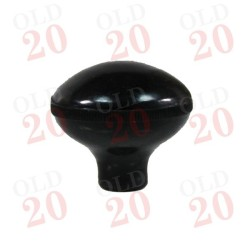•# Gear Lever Knob

1100004

£9.00
Tax included
QuantitySecure Online Ordering

All our pages are encrypted for your securityWorldwide Delivery

We ship nearly all the parts we supply worldwide!12 Month Warranty

All parts covered by a 12 month warranty

1100004

Section
Transmission

### Specific References

• Tractors < Fordson and Ford < Fordson < Standard N

• Tractors < Fordson and Ford < Fordson < E27N (TVO)

• Tractors < Fordson and Ford < Fordson < E27N (P6)

• Tractors < Fordson and Ford < Fordson < E1A Major

• Tractors < Fordson and Ford < Fordson < Power Major

• Tractors < Fordson and Ford < Fordson < Super Major

• Tractors < Fordson and Ford < 1000 Series < 2000

• Tractors < Fordson and Ford < 1000 Series < 3000

• Tractors < Fordson and Ford < 1000 Series < 4000

• Tractors < Fordson and Ford < 1000 Series < 5000

• Tractors < John Deere < 30 < 1030

• Tractors < John Deere < 30 < 1130

• Tractors < John Deere < 30 < 1630

• Tractors < John Deere < 30 < 1830

• Tractors < John Deere < 30 < 2030

• Tractors < John Deere < 30 < 2130

• Tractors < John Deere < 30 < 3030

• Tractors < John Deere < 30 < 3130

• Tractors < John Deere < 30 < 830

• Tractors < John Deere < 30 < 930

• Tractors < John Deere < 40 < 1040

• Tractors < John Deere < 40 < 1140

• Tractors < John Deere < 40 < 1640

• Tractors < John Deere < 40 < 1840

• Tractors < John Deere < 40 < 2040

• Tractors < John Deere < 40 < 2040S

• Tractors < John Deere < 40 < 2140

• Tractors < John Deere < 40 < 3040

• Tractors < John Deere < 40 < 3140

• Tractors < John Deere < 40 < 3340

• Tractors < John Deere < 40 < 3640

• Tractors < John Deere < 40 < 3640S

• Tractors < John Deere < 40 < 4040

• Tractors < John Deere < 40 < 4240

• Tractors < John Deere < 40 < 4240S

• Tractors < John Deere < 40 < 4440

• Tractors < John Deere < 40 < 840

• Tractors < John Deere < 40 < 940

• Tractors < John Deere < 50 < 1350

• Tractors < John Deere < 50 < 1550

• Tractors < John Deere < 50 < 1750

• Tractors < John Deere < 50 < 1950

• Tractors < John Deere < 50 < 2250

• Tractors < John Deere < 50 < 2450

• Tractors < John Deere < 50 < 2650

• Tractors < John Deere < 50 < 2850

• Tractors < John Deere < 50 < 3050

• Tractors < John Deere < 50 < 3150

• Tractors < John Deere < 50 < 3350

• Tractors < John Deere < 50 < 4350

• Tractors < Massey Ferguson < Pre-100 Series < 35

• Tractors < Massey Ferguson < Pre-100 Series < 35X

• Tractors < Massey Ferguson < Pre-100 Series < 65

• Tractors < Massey Ferguson < 100 Series < 135

• Tractors < Massey Ferguson < 100 Series < 148

• Tractors < Massey Ferguson < 100 Series < Various MF100 Series < 155

• Tractors < Massey Ferguson < 100 Series < 158

• Tractors < Massey Ferguson < 100 Series < 165

• Tractors < Massey Ferguson < 100 Series < 168

• Tractors < Massey Ferguson < 100 Series < Various MF100 Series < 168S

• Tractors < Massey Ferguson < 100 Series < 175

• Tractors < Massey Ferguson < 100 Series < 178

• Tractors < Massey Ferguson < 100 Series < 185

• Tractors < Massey Ferguson < 100 Series < 188

• Tractors < Massey Ferguson < 200 Series < 230

• Tractors < Massey Ferguson < 200 Series < 240

• Tractors < Massey Ferguson < 200 Series < 250

• Tractors < Massey Ferguson < 200 Series < Various < 253

• Tractors < Massey Ferguson < 200 Series < 265

• Tractors < Massey Ferguson < 200 Series < 275

• Tractors < Massey Ferguson < 200 Series < Various < 285

• Tractors < Massey Ferguson < 200 Series < 290

• Tractors < Massey Ferguson < 500 < 550

• Tractors < Massey Ferguson < 500 < 565

• Tractors < Massey Ferguson < 500 < 575

• Tractors < Massey Ferguson < 500 < 590

• Tractors < Massey Ferguson < 500 < 595

• Tractor Sections < Transmission < Selector

13418B, 765961M1, 237487, 87762999, 87771084, BB7213A, 83901458,